# A Simple Plagiarism Rate Checker Using Rabin-Karp String Matching Algorithm in Python and Golang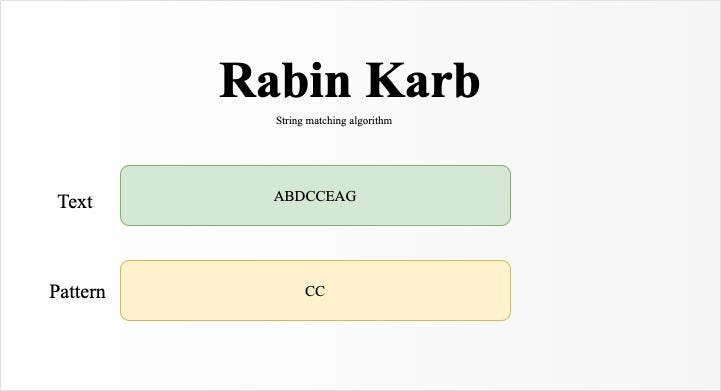Plagiarism checker is the process of locating instances of plagiarism in a document. According to Oxford Dictionary of English, plagiarism is “the practice of taking someone else’s work or ideas and passing them off as one’s own” without proper citations. The widespread use of computers and the advent of the Internet have made it easier to plagiarize the work of others and get away with it, but with the help of a computer-aided plagiarism detector, we can determine the plagiarism level of two documents using the Rabin Karp algorithm.

### What is Rabin Karp?

Rabin Karp algorithm is a string matching algorithm and this algorithm makes use of hash functions and the rolling hash technique, A hash function is essentially a function that map data of arbitrary size to a value of fixed size while A rolling hash allows an algorithm to calculate a hash value without having to rehash the entire string, this allows a new hash to be computed very quickly. We need to take note of two sets of data which are pattern and text, the pattern represents the string we are trying to match in the text.

The Rabin-Karp algorithm is an improved version of the brute force approach which compares each character of pattern **with each character of **text **and has a time complexity of **O(mn)

Major features

• uses a hashing function techniques and rolling hash;
• preprocessing phase in O(m) time complexity and constant space;
• searching phase in O(nm) time complexity;
• O(n+m) expected running time.

## How it works

Given a pattern “cc” and a text **“abdcceag”, **I will demonstrate how the Rabin Karp algorithm works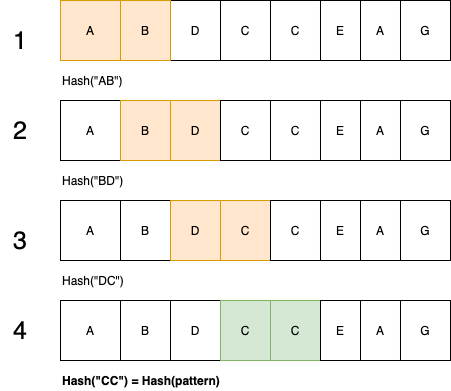As we iterate through the text we will generate the hash value for the current window which is of length pattern and compare it with the hash value of the pattern, if they don’t match, we move to the next window to generate the next hash value using the rolling hash technique. NB: subsequent hashes are computed from the previous hash, E.G “BD” is computed from “AB” E.T.C.

and finally, we found a match in the 4th window. So how can this simple technique be used in plagiarism detection in documents? well, I found two interesting research papers that did justice to that and this article further explains that with code implementations.

### Hash Functions

The hash function is a function that determines the feature value of a particular syllable fraction or word in the document. It converts each string into a number, called a hash value. Rabin-Karp algorithm determines hash value based on the same word K-Gram.

K-grams are k-length subsequences of a string, it can be 1,2,3,4 E.T.C. These are mostly used for spelling correction, but in this case, we are using k-gram to determine plagiarism pattern between two documents. K-gram is going to replace our pattern length in figure 1. **A good hash function is important in order to prevent multiple words from having the same hash value which is popularly known as **spurious hit and a bad hashing will invalidate our solution in the long run.

We will be using a **modular arithmetic hash function with a **technique called rolling hash where the input is hashed in a window that moves or iterate through the input in this article.

### Modular arithmetic hash function:

We compute our string using a modular arithmetic hash function using the formula below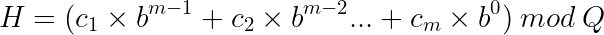Where:

• Given that every character has ASCII code that maps to it E.G A => 1, B => 2
• c1, c2, c3…..ck represents the ASCII code of each character in a string
• m represents the k-gram or number of characters there are in the string we are comparing
• where b is a constant
• where Q is modulo.

Example:

Given a pattern “cc” and a text “abdcceag”, **let us find the hash of pattern **“cc”

Where: b = 26, m = 2, Q = 5807 H("cc")= ((3×261 ) % 5807 + (3×260) ) % 5807 H("cc") = 81

### Rolling Hash

As discussed earlier, the rolling hash allows us to compute a new hash rapidly using the previous hash. We will compute our new hash using the formula below: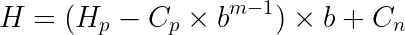Example:

Given the text “abdcceag”, **let’s find the hash of window **“ab” and “bd” **using the **Modular arithmetic hash function and rolling hash technique.

Where: b **= 26, m = 2, **Q = 5807 H(“ab”)= ((1×261 ) % 5807 + (2×260) ) % 5807 H(“ab”) = 28 H(“bd”) = ((H(“ab”) — H(“a”) )* b + h(“d”)) % Q H(“bd”) = ((28-26) * 26 + 4) % 5807 H(“bd”) = 56

## Solution

We understand how Rabin Karp works, now it’s time to move on to the actual implementation, this process is going to involve **four(4) **different steps which include: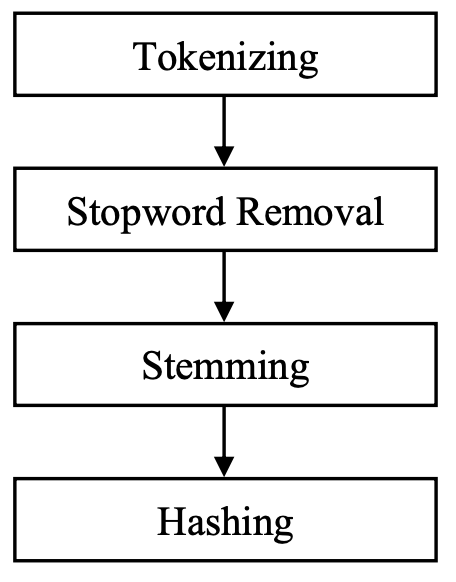### step 1: Tokenizing

Tokenizing is essentially splitting a phrase, sentence, paragraph, or an entire text document into smaller units, such as individual words or terms. Each of these smaller units are called tokens.

### step 2: Stopword Removal

The process of removing basic words that always exist in the document such as: because, with, and, or, not, and others.

### step 3: Stemming

Stemming is the process of reducing a word to its word stem that affixes to suffixes and prefixes or to the roots of words

known as a lemma in NLP. E.g car, cars, car’s, cars’ has the stem car

Code Implementation

Python

The prepare_content() handles stemming, tokenizing, and stopword removal, using natural language tool kit library (nltk)

src/process_content.py
``````import nltk
from nltk.corpus import stopwords
from nltk.tokenize import word_tokenize
from nltk.stem import PorterStemmer
from nltk.stem import LancasterStemmer

class PlagiarismChecker:    def prepare_content(self, content):        # STOP WORDS        stop_words = set(stopwords.words('english'))        # TOKENIZE        word_tokens = word_tokenize(content)        filtered_content = []        # STEMMING        porter = PorterStemmer()        for w in word_tokens:            if w not in stop_words:                w = w.lower()                word = porter.stem(w)                filtered_content.append(word)        return filtered_content``````

Golang

Golang is a new programming language that has less support for natural language processing but I found some interesting libraries that implements stemming and tokenization pretty well. Check the code below but please endeavor to check the github repo for better context

src/process_content.go
``````package main

import (
prose "github.com/jdkato/prose/v2"
porterstemmer "github.com/reiver/go-porterstemmer"
)

type PlagiarismChecker struct {
HashTable map[string][]int
KGram     int
}

// PrepareContent, prepares content by removing stopwords, steemming and tokenizing
func (pc PlagiarismChecker) PrepareContent(content string) string {	// TOKENIZE	doc, err := prose.NewDocument(content)	if err != nil {		log.Fatal(err)	}	data := []string{}	// Iterate over the doc's tokens:	for _, tok := range doc.Tokens() {		// STOP WORD REMOVAL		if !Contains(stopwords, tok.Text) {			// STEMMING			stem := porterstemmer.StemString(tok.Text)			data = append(data, stem)		}	}	return strings.Join(data[:], "")}``````

### step 4: Hashing

This step generates the hash value for the content in our document using the Rabin Karp algorithm with a specified K-gram value that determines the length of the string we need for each window after it has undergone the 3 steps listed above.

### Implementation of Rabin Karp algorithm

Using the rolling hash technique and modular hash function

### Python

src/rabin_karp.py
``````class rolling_hash:
def __init__(self, text, patternSize):
self.text = text
self.patternSize = patternSize
self.base = 26
self.window_start = 0
self.window_end = 0
self.mod = 5807
self.hash = self.get_hash(text, patternSize)

def get_hash(self, text, patternSize):
hash_value = 0
for i in range(0, patternSize):
hash_value += (ord(self.text[i]) - 96)*(self.base**(patternSize - i -1)) % self.mod

self.window_start = 0
self.window_end =  patternSize

return hash_value
def next_window(self):
if self.window_end <= len(self.text) - 1:
self.hash -= (ord(self.text[self.window_start]) - 96)*self.base**(self.patternSize-1)
self.hash *= self.base
self.hash += ord(self.text[self.window_end])- 96
self.hash %= self.mod
self.window_start += 1
self.window_end += 1
return True
return False
def current_window_text(self):
return self.text[self.window_start:self.window_end]

def checker(text, pattern):
if text == "" or pattern == "":
return None
if len(pattern) > len(pattern):
return None

text_rolling = rolling_hash(text, len(pattern))
pattern_rolling = rolling_hash(pattern, len(pattern))

for _ in range(len(text) -  len(pattern) - 1):
if text_rolling.hash == pattern_rolling.hash:
return "Found"
text_rolling.next_window()

if __name__ == "__main__":
print(checker("abcdefgh", "a"))``````

### Golang

src/rabin_karp.go
``````package main

import (
"fmt"
"math"
"strings"
)

type RabinKarp struct {
Base        int
Text        string
PatternSize int
Start       int
End         int
Mod         int
Hash        int
}

func NewRabinKarp(text string, patternSize int) *RabinKarp {
rb := &RabinKarp{
Base:        26,
PatternSize: patternSize,
Start:       0,
End:         0,
Mod:         5807,
Text:        text,
}

rb.GetHash()

return rb
}

//GetHash creates hash for the first window
func (rb *RabinKarp) GetHash() {
hashValue := 0
for n := 0; n < rb.PatternSize; n++ {
runeText := []rune(rb.Text)
value := int(runeText[n])
mathpower := int(math.Pow(float64(rb.Base), float64(rb.PatternSize-n-1)))
hashValue = Mod((hashValue + (value-96)*(mathpower)), rb.Mod)
}
rb.Start = 0
rb.End = rb.PatternSize

rb.Hash = hashValue
}

// NextWindow returns boolean and create new hash for the next window
// using rolling hash technique
func (rb *RabinKarp) NextWindow() bool {
if rb.End <= len(rb.Text)-1 {
mathpower := int(math.Pow(float64(rb.Base), float64(rb.PatternSize-1)))

rb.Hash -= (int([]rune(rb.Text)[rb.Start]) - 96) * mathpower
rb.Hash *= rb.Base
rb.Hash += int([]rune(rb.Text)[rb.End] - 96)
rb.Hash = Mod(rb.Hash, rb.Mod)
rb.Start++
rb.End++
return true
}
return false
}

// CurrentWindowText return the current window text
func (rb *RabinKarp) CurrentWindowText() string {
return rb.Text[rb.Start:rb.End]
}

func Checker(text, pattern string) string {
textRolling := NewRabinKarp(strings.ToLower(text), len(pattern))
patternRolling := NewRabinKarp(strings.ToLower(pattern), len(pattern))

for i := 0; i <= len(text)-len(pattern)+1; i++ {
fmt.Println(textRolling.Hash, patternRolling.Hash)
if textRolling.Hash == patternRolling.Hash {
return "Found"
}
textRolling.NextWindow()
}
}

func main() {
text := "ABDCCEAGmsslslsosspps"
pattern := "agkalallaa"
fmt.Println(Checker(text, pattern))
}``````

### Python

The below code makes use of the above-implemented Rabin Karp algorithm to calculate and generate the hash for our document content. Take note ofcalculatehash() function and kgram value, in this case, we are using 5 as our k-gram

src/calculate_hash.py
``````import nltk
from nltk.corpus import stopwords
from nltk.tokenize import word_tokenize
from nltk.stem import PorterStemmer
from nltk.stem import LancasterStemmer
import rabin_karp
import numpy as np
from os.path import dirname, join

class PlagiarismChecker:
def __init__(self, file_a, file_b):
self.valid = False
self.file_a = file_a
self.file_b = file_b
self.hash_table = {"a": [], "b": []}
self.k_gram = 5
content_a = self.get_file_content(self.file_a)
content_b = self.get_file_content(self.file_b)
self.calculate_hash(content_a, "a")
self.calculate_hash(content_b, "b")

# calaculate hash value of the file content   # and add it to the document type hash table    def calculate_hash(self, content, doc_type):       text = self.prepare_content(content)       text = "".join(text)       text = rabin_karp.rolling_hash(text, self.k_gram)       for _ in range(len(content) - self.k_gram + 1):           self.hash_table[doc_type].append(text.hash)           if text.next_window() == False:               break   # get content from file
def get_file_content(self, filename):
file = open(filename, 'r+', encoding="utf-8")

# Prepare content by stemming, tokenizing and removing stopwords.
def prepare_content(self, content):
# STOP WORDS
stop_words = set(stopwords.words('english'))
# TOKENIZE
word_tokens = word_tokenize(content)
filtered_content = []
# STEMMING
porter = PorterStemmer()
for w in word_tokens:
if w not in stop_words:
w = w.lower()
word = porter.stem(w)
filtered_content.append(word)

return filtered_content``````

### Golang

Take note of the CalculateHash() function and KGram value

src/calculate_hash.go
``````package main

import (
"fmt"
"io/ioutil"
"log"
"path"
"path/filepath"

"strings"

prose "github.com/jdkato/prose/v2"
porterstemmer "github.com/reiver/go-porterstemmer"
)

type PlagiarismChecker struct {
HashTable map[string][]int
KGram     int
}

var (
_, currentPath, _, _ = runtime.Caller(0)
)

func NewPlagiarismChecker(fileA, fileB string) *PlagiarismChecker {
checker := &PlagiarismChecker{
KGram:     5,
HashTable: make(map[string][]int),
}
checker.CalculateHash(checker.GetFileContent(fileA), "a")
checker.CalculateHash(checker.GetFileContent(fileB), "b")

return checker
}

// PrepareContent, prepares content by removing stopwords, steemming and tokenizing
func (pc PlagiarismChecker) PrepareContent(content string) string {

// TOKENIZE
doc, err := prose.NewDocument(content)
if err != nil {
log.Fatal(err)
}
data := []string{}
// Iterate over the doc's tokens:

for _, tok := range doc.Tokens() {
// STOP WORD REMOVAL
if !Contains(stopwords, tok.Text) {
// STEMMING
stem := porterstemmer.StemString(tok.Text)
data = append(data, stem)
}
}
return strings.Join(data[:], "")
}

//  CalculateHash calaculates the hash value of the file content// and add it to the document type hash tablefunc (pc PlagiarismChecker) CalculateHash(content, docType string) {	text := pc.PrepareContent(content)	fmt.Println(text)	textRolling := NewRabinKarp(strings.ToLower(text), pc.KGram)	for i := 0; i <= len(text)-pc.KGram+1; i++ {		if len(pc.HashTable[docType]) == 0 {			pc.HashTable[docType] = []int{textRolling.Hash}		} else {			pc.HashTable[docType] = append(pc.HashTable[docType], textRolling.Hash)		}		if textRolling.NextWindow() == false {			break		}	}	fmt.Println(pc.HashTable)}
// GetFileContent get content from file
func (pc PlagiarismChecker) GetFileContent(filename string) string {
if err != nil {
panic(err)
}

return string(data)
}``````

### Rate Calculation

We compute Plagiarism Rate using the formula belowWhere:

• P is the Plagiarism Rate
• **SH **is the Identical Hash
• THA is the Total Hash in Document A
• THB is the Total Hash in Document B

### Final Code *(Python)*

Take note of the calculateplagiarismrate() function, we computed this function using the formula given above and we calculated our identical hash using numpy intersect1d() function

src/calculate_hash.go
``````import nltk
from nltk.corpus import stopwords
from nltk.tokenize import word_tokenize
from nltk.stem import PorterStemmerfrom nltk.stem import LancasterStemmerimport rabin_karpimport numpy as np
from os.path import dirname, join

class PlagiarismChecker:
def __init__(self, file_a, file_b):
self.file_a = file_a
self.file_b = file_b
self.hash_table = {"a": [], "b": []}
self.k_gram = 5
content_a = self.get_file_content(self.file_a)
content_b = self.get_file_content(self.file_b)
self.calculate_hash(content_a, "a")
self.calculate_hash(content_b, "b")

# calaculate hash value of the file content
# and add it to the document type hash table
def calculate_hash(self, content, doc_type):
text = self.prepare_content(content)
text = "".join(text)

text = rabin_karp.rolling_hash(text, self.k_gram)
for _ in range(len(content) - self.k_gram + 1):
self.hash_table[doc_type].append(text.hash)
if text.next_window() == False:
break

def get_rate(self):
return self.calaculate_plagiarism_rate(self.hash_table)

# calculate the plagiarism rate using the plagiarism rate formula
def calaculate_plagiarism_rate(self, hash_table):
th_a = len(hash_table["a"])
th_b = len(hash_table["b"])
a = hash_table["a"]
b = hash_table["b"]
sh = len(np.intersect1d(a, b))
print(sh, a, b)

# Formular for plagiarism rate
# P = (2 * SH / THA * THB ) 100%
p = (float(2 * sh)/(th_a + th_b)) * 100
return p

# get content from file
def get_file_content(self, filename):
file = open(filename, 'r+', encoding="utf-8")

# Prepare content by removing stopwords, steemming and tokenizing
def prepare_content(self, content):
# STOP WORDS
stop_words = set(stopwords.words('english'))
# TOKENIZE
word_tokens = word_tokenize(content)
filtered_content = []
# STEMMING
porter = PorterStemmer()
for w in word_tokens:
if w not in stop_words:
w = w.lower()
word = porter.stem(w)
filtered_content.append(word)

return filtered_content

current_dir = dirname(__file__)
checker = PlagiarismChecker(
join(current_dir, "./document_a.txt"),
join(current_dir, "./document_b.txt")
)
print('The percentage of plagiarism held by both documents is  {0}%'.format(
checker.get_rate()))``````

### Final code *(Golang)*

Take note of the CalculatePlagiarismRate() function.

src/calculate_hash.go
``````package main

import (
"fmt"
"io/ioutil"
"log"
"path"
"path/filepath"
"reflect"
"runtime"

"strings"

prose "github.com/jdkato/prose/v2"
porterstemmer "github.com/reiver/go-porterstemmer"
)

type PlagiarismChecker struct {
HashTable map[string][]int
KGram     int
}

var (
_, currentPath, _, _ = runtime.Caller(0)
)

func NewPlagiarismChecker(fileA, fileB string) *PlagiarismChecker {
checker := &PlagiarismChecker{
KGram:     5,
HashTable: make(map[string][]int),
}
checker.CalculateHash(checker.GetFileContent(fileA), "a")
checker.CalculateHash(checker.GetFileContent(fileB), "b")

return checker
}

// PrepareContent, prepares content by removing stopwords, steemming and tokenizing
func (pc PlagiarismChecker) PrepareContent(content string) string {

// TOKENIZE
doc, err := prose.NewDocument(content)
if err != nil {
log.Fatal(err)
}
data := []string{}
// Iterate over the doc's tokens:

for _, tok := range doc.Tokens() {
// STOP WORD REMOVAL
if !Contains(stopwords, tok.Text) {
// STEMMING
stem := porterstemmer.StemString(tok.Text)
data = append(data, stem)
}
}
return strings.Join(data[:], "")
}

//  CalculateHash calaculates the hash value of the file content
// and add it to the document type hash table
func (pc PlagiarismChecker) CalculateHash(content, docType string) {
text := pc.PrepareContent(content)
fmt.Println(text)

textRolling := NewRabinKarp(strings.ToLower(text), pc.KGram)

for i := 0; i <= len(text)-pc.KGram+1; i++ {
if len(pc.HashTable[docType]) == 0 {
pc.HashTable[docType] = []int{textRolling.Hash}
} else {
pc.HashTable[docType] = append(pc.HashTable[docType], textRolling.Hash)
}
if textRolling.NextWindow() == false {
break
}
}

fmt.Println(pc.HashTable)
}

func (pc PlagiarismChecker) GetRate() float64 {
return pc.CalculatePlagiarismRate()
}

// GetFileContent get content from file
func (pc PlagiarismChecker) GetFileContent(filename string) string {
if err != nil {
panic(err)
}

return string(data)
}

// CalculatePlagiarismRate calculates the plagiarism rate using the plagiarism rate formula
func (pc PlagiarismChecker) CalculatePlagiarismRate() float64 {

THA := len(pc.HashTable["a"])
THB := len(pc.HashTable["b"])
intersect := Intersect(pc.HashTable["a"], pc.HashTable["b"])
SH := reflect.ValueOf(intersect).Len()

// Formular for plagiarism rate
// P = (2 * SH / THA + THB ) 100%
fmt.Println(SH, THA, THB)
p := float64(2*SH) / float64(THA+THB)
return float64(p * 100)
}

func main() {
//get root
Root := filepath.Join(filepath.Dir(currentPath), "../")
docsPath := path.Join(Root, "/docs/")
checker := NewPlagiarismChecker(
path.Join(docsPath, "/document_a.txt"),
path.Join(docsPath, "/document_b.txt"),
)
fmt.Printf("The percentage of plagiarism held by both documents is  %f", checker.GetRate())
}``````

NB: “The length of K-Gram is the determinant of the plagiarism level. Determining the proper length of K-Gram, improves our plagiarism level results. The results will vary foreach K-Gram value.”

### Demo

Given document A

``The process of searching for a job can be very stressful, but it doesn’t have to be. Start with a well-written resume that has appropriate keywords for your occupation. Next, conduct a targeted job search for positions that meet your needs.``

…and document B

``Looking for a job can be very stressful, but it doesn’t have to be. Begin by writing a good resume with appropriate keywords for your occupation. Second, target your job search for positions that match your needs.``

….after the computation of both documents in python, The percentage of plagiarism held by both documents is 50.76142131979695% while **in golang i got **53.731343%.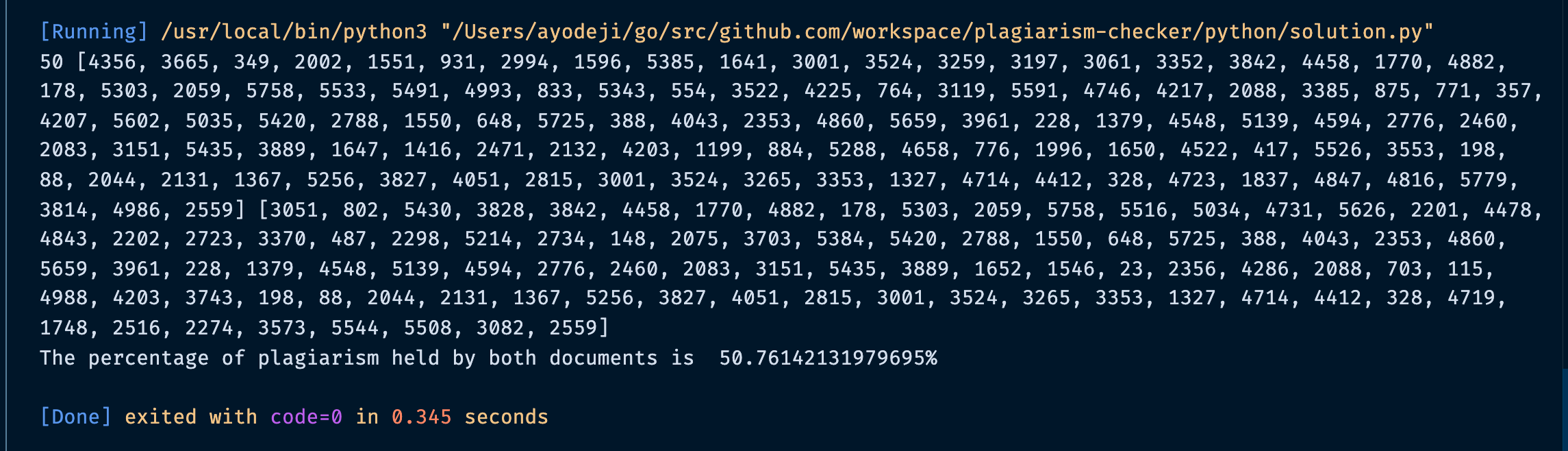## Time Complexity

The Rabin-Karp algorithm has the complexity of O(nm) for the worst case, O(n + m) for the best case where n **is the length of the text, while **m is the length of the pattern. So where is it compared to brute-force matching? Well, brute force matching complexity is O(nm), so as it seems there’s not much of a gain in performance. However, it’s considered that Rabin-Karp’s complexity is O(n+m) in practice with an efficient hash function and that makes it a bit faster.

## Conclusion

Detecting the rate of plagiarism in a document is dependent on the value of k-gram and the calculation of hash value greatly affects the result of this algorithm. Higher K-Gram values tend to have a low level of similarity, while lower K-Gram values increase the percentage of similarity, and also , agood hash function is crucial in order to prevent spurious hit, to be honest, the modular hash function is not the best, check out this article for other interesting techniques to improve our hash function. This solution is not the best but I believe we have been able to demonstrate an interesting solution on how this can work in real-life applications